Disclosure: This post may contain affiliate links, meaning when you click the links and make a purchase, we receive a commission.

# GRG Nonlinear Multistart & Excel Evolutionary Solver (Explained)!

Previously I have written an article on how to rate sports team using GRG Nonlinear engine, today I’d like to continue to discuss GRG Nonlinear Multistart and Excel evolutionary solver engine in more details.

Stay tuned…

## 1. Understand GRG Nonlinear Engine

If the references to changing cells in both target cell and constraints are in the (Changing cell)*(Constant) form, you have a linear model. Otherwise, you have a nonlinear model. For example, if x and y are changing cells, references like those in Figure 1 in your target cell or constraint can make your model nonlinear and you can choose GRG Nonlinear engine.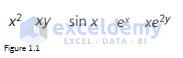Figure 1.1

The first two graphs in Figure 1.2 are for functions y = -x^2+4x+2 and y = x^2 respectively. For left panel, you can see that there is a point (x = 2) at which the slope of the function is 0. And for the middle panel, there is also a point at which the slope of the function is 0. You can see that when the slope of the function is 0, the function will be maximized (left panel) or minimized (middle panel).  In fact, this is how the GRG Nonlinear engine solves the problem – try to find a point at which the slope of the function is 0.

Unfortunately, many functions cannot be maximized or minimized simply by locating a point where the function’s slope is 0. Let’s look at the right panel of Figure 1.2. The function has more than one peak and there are 7 points at which the slope of the function is 0. If Excel starts near x = 5, Excel will return a solution of x = 5. Obviously, this is incorrect. Similarly, x = -15 is also not the right solution. Only when Excel starts near x = 1, you will get the right solution.

In most problems with more than one changing cell, Excel does not know which point is the good starting point. Fortunately, Excel provides a Multistart option. You can select Multistart after choosing Options and then clicking the GRG Nonlinear tab. When the Multistart option is selected, Excel chooses many starting points and finds the best solution.

And there are also some problems in which target cell, constraints, or both use non-smooth function such as MAX, MIN, ABS, IF, SUMIF, COUNTIF, SUMIFS, COUNTIFS, GRG engine cannot be used. Look at both the left panel and right panel of Figure 1.4, there are no points at which function’s slope is 0. For this kind of problems, we need to use the Evolutionary engine.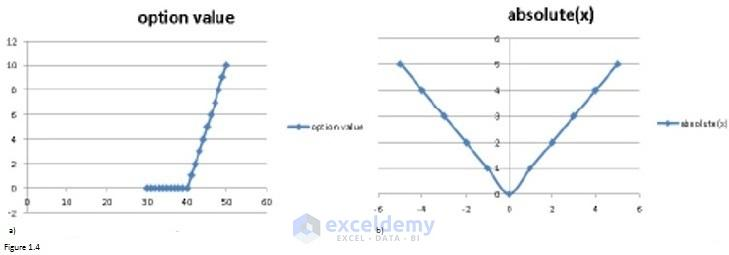Figure 1.4

## 2. Problem 1: How to locate a single warehouse to minimize the total distance that packages are shipped?

Suppose that an internet shipping company want to build a single warehouse, where the warehouse should be located so that the total distance that packages are shipped can be minimized? The number of shipments (in thousands) made each year to various cities is shown in the following table.

 City Latitude Longitude Shipments (in thousands) New York 40.7 73.9 15 Boston 42.3 71 8 Philadelphia 40 75.1 10 Charlotte 35.2 80.8 6 Atlanta 33.8 84.4 11 New Orleans 30 89.9 8 Miami 25.8 80.2 13 Dalla 32.8 96.8 10 Houston 29.8 95.4 12 Chicago 41.8 87.7 14 Detroit 42.4 83.1 11 Cleveland 41.5 81.7 8 Indy 39.8 86.1 7 Denver 39.8 105 8 Minneapolis 45 93.3 9 Phoenix 33.5 112 11 Salt Lake City 40.8 112 10 LA 34.1 118 18 SF 37.8 123 12 SD 32.8 117 10 Seattle 41.6 112 13

### 2.1 Equation to compute the approximate distance between two sites

Figure 2.1 shows formula which gives the approximate distance between two US cities having a latitude and longitude given by (Lat1, Long1) and (Lat2, Long2).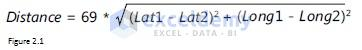Figure 1.2

Read more: How to Use Solver in Excel (Solving Linear Programming Problems)!

### 2.2 Set up Model

Let’s take cells J2 and J3 as By Changing cells and they include latitude and longitude of the warehouse respectively. The distance between New York and warehouse can be calculated by entering the formula “=69*SQRT((C3-\$J\$2)^2+(D3-\$J\$3)^2)” into cell F3. By copying this formula into cells from F4 through F23, we can get distances between the warehouse and other cities. Formula “=E3*F3” in cell G3 can be used to compute shipped distances between New York and Warehouse. Similarly, copying this formula into range G4: G23, we can calculate shipped distances for other cities. Sum all the shipped distances by filling formula “=SUM(G3: G23)” in cell J4, our objective – total shipped distances – can be computed. Thus, cell J4 is our Target cell.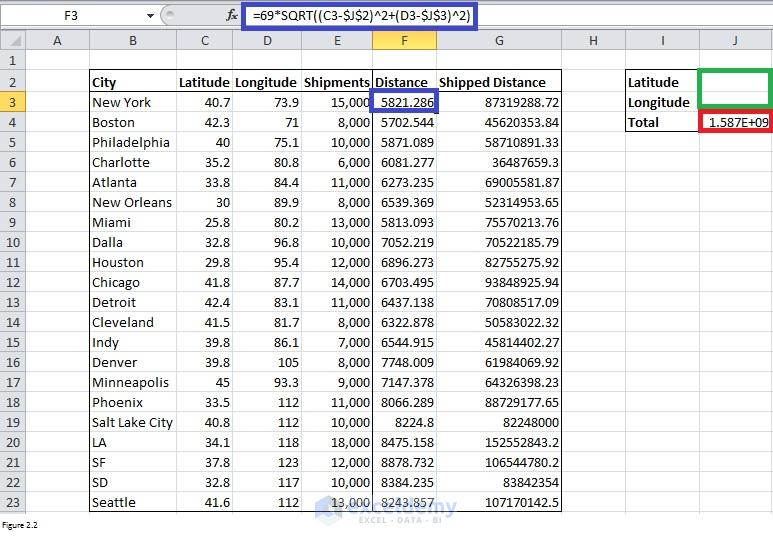Figure 2.2

### 2.3 Use Excel Solver to solve a problem

Click on Solver in Analysis group to open Solver Parameters dialog box. Fill the Solver Parameters dialog box as shown in Figure 2.3. Per the equation in section 2.1, GRG Nonlinear engine should be selected for this problem. In order to make sure that the warehouse is north of the equator, west of Greenwich, England, and east of Anchorage, Alaska, we need to set up two constraints. Latitude should be between 0 and 90 degrees while longitude should be between 0 and 150.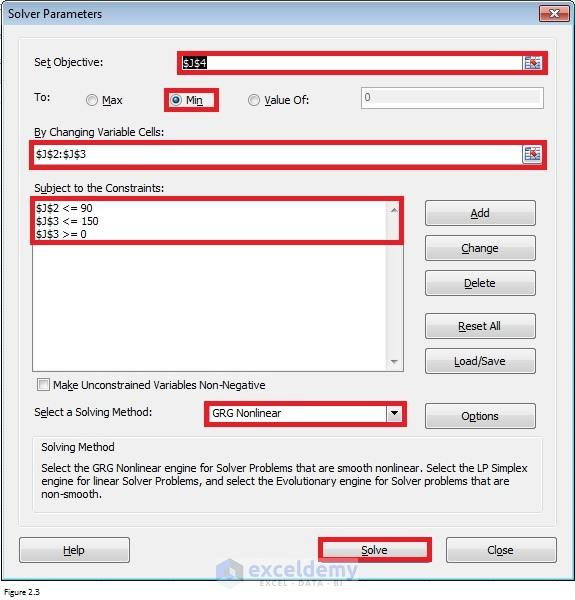Figure 2.3

After you click Solve, you’ll find that the warehouse should be located at 36.90 degrees latitude and 92.48 degrees longitude.

## 3. Problem 2: How to locate two warehouses to minimize the total distance that packages are shipped?

Still for above same problem, what if the company would like to build two warehouses? How should the company locate those two warehouses?

Read More: Deal with Sequencing Problems Using Excel Solver!

### 3.1 Set up Model

There are two warehouses now. Cells L2 and M2 are for the latitude of warehouse 1 and warehouse 2. Cells L3 and M3 include longitude of warehouse 1 and warehouse 2. These 4 cells are our By Changing Cells.

We can use the same way as that in section 2.2 to compute distances between each city and each warehouse. For example, formula “=69*SQRT((C4-\$L\$2)^2+(D4-\$L\$3)^2)” in cell F4 returns distance between Boston and Warehouse 1 while formula “=69*SQRT((D4-\$M\$2)^2+(E4-\$M\$3)^2)” in cell G4 returns distance between Boston and Warehouse 2. Because the shipments to each city will be sent from the closer warehouse, we need to compute the distance of each city to the closer ware­house by copying the formula “=MIN(F3, G3)” from H3 to H84: H23. Next, use the similar method as that in section 2.2 to get total distance traveled by shipments for each city. Our target cell L4 displays total distances returned by formula “=SUM(I3: I23)”.

### 3.2 use Excel Solver to solve the problem

Click on Solver in Analysis group to open Solver Parameters dialog box. Fill the Solver Parameters dialog box as shown in Figure 3.2. Since we suspect that there are multiple peaks for the function, I selected GRG MultiStart option. Please note that bounds (constraints) for By Changing Cells should always be set if you use GRG MultiStart.  The bounds here for this case is the same as that in section 2.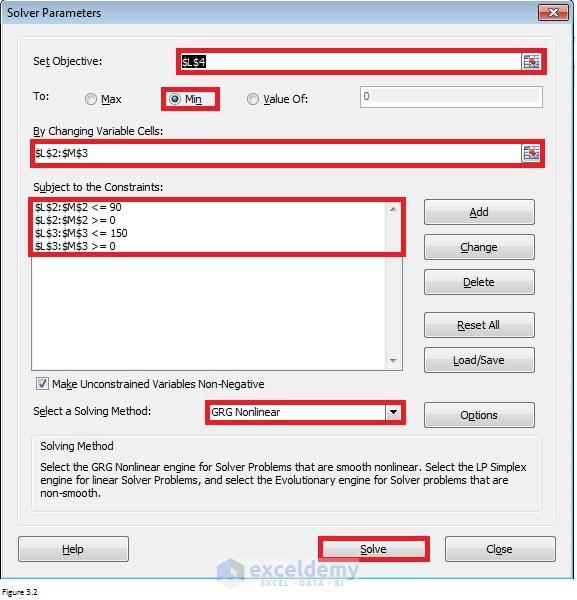Figure 3.2

After clicking on Solve, latitude, and longitude for Warehouse 1 returned by Excel is 38.1 and 84.0. Latitude and longitude for Warehouse 2 are 35.7 and 115.7.

## Remark

To confirm that Solver found the optimal solution, you can select Evolutionary engine and run Solver again. In our case, you will find that the current solution is already the best one.

Suppose that Solver recommends a longitude near 150 degrees, you should relax the bound to avoid a mistake.

Financial Planning with Excel Solver [2 Case Studies]

Sequencing problem using Johnson’s algorithm of scheduling n-jobs on 2-machines [Sol]

GRG-Multistart-and-Evolutionary-Excel-Solver-Engines.xlsx

I am from China and this photo was taken in a classical garden. There are many similar gardens in China, attracting a lot of visitors every year, especially in spring and summer.

I was major in Biotechnology. But I took a job as an SAS programmer because I prefer programming. Besides SAS, I also learned Excel VBA in my spare time. It is fantastic to be able to manipulate data, files and even to interact with the internet via programming. This will save me a lot of time. I am keen to learn new things.

1 Comment
1. ReplyOct 24, 2016 at 8:35 PM

Nice post!

It would be good to see a case study of how to use the evolutionary solver for binary problems, such as the assignment problem with more than 30 decision variables.